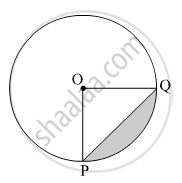Advertisement Remove all ads

# In the given figure, if O is the center of the circle, PQ is a chord. ∠ POQ = 90°, area of the shaded region is 114 cm2, find the radius of the circle. π = 3.14) - Geometry

Advertisement Remove all ads
Advertisement Remove all ads
Advertisement Remove all ads
Sum

A chord PQ of a circle with a radius of cm subtends an angle of 60° with the center of the circle. Find the area of the minor as well as the major segment. ( $\pi$ = 3.14,  $\sqrt{3}$ = 1.73)

Advertisement Remove all ads

#### Solution

The radius of the circle, r = 15 cm
Let O be the center and PQ be the chord of the circle.∠POQ = θ = 60º
Area of the minor segment = Area of the shaded region

$= r^2 \left( \frac{\pi\theta}{360°} - \frac{\sin\theta}{2} \right)$
$= \left( 15 \right)^2 \times \left( \frac{3 . 14 \times 60° }{360° } - \frac{\sin60° }{2} \right)$
$= 225 \times \frac{3 . 14}{6} - 225 \times \frac{\sqrt{3}}{4}$
$= 117 . 75 - 97 . 31$
$= 20 . 44 {cm}^2$

Now,
Area of the circle =

$\pi r^2 = 3 . 14 \times \left( 15 \right)^2 = 3 . 14 \times 225$  = 706.5 cm2
∴ Area of the major segment = Area of the circle − Area of the minor segment = 706.5 − 20.44 = 686.06 cm2
Thus, the areas of the minor segment and major segment are 20.44 cm2 and 686.06 cm2, respectively.
Concept: Areas of Sector and Segment of a Circle
Is there an error in this question or solution?

#### APPEARS IN

Balbharati Mathematics 2 Geometry 10th Standard SSC Maharashtra State Board
Chapter 7 Mensuration
Practice set 7.4 | Q 5 | Page 160
Advertisement Remove all ads
Share
Notifications

View all notifications

Forgot password?